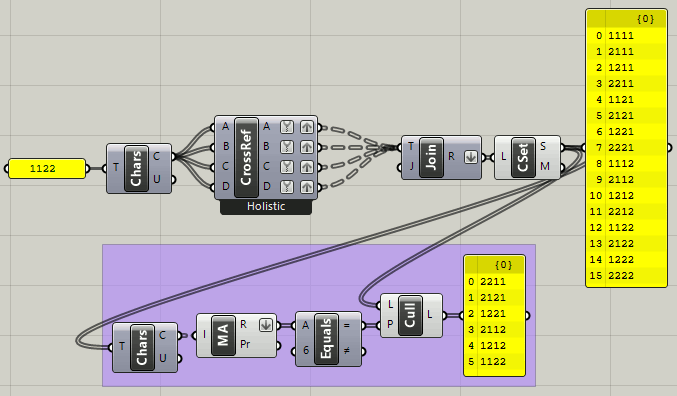# Creating a matrix of all possible outputs of a definition

Hello,

I have created a definition that uses lists to set dimensions of a model for 3D printing.

For example: if I had a rectangular extrusion, one list would correspond to the width of the rectangle (10, 20, or 30 mm wide), another list would set the height of the rectangle (10mm, 20mm, or 30mm) and another list would set the depth of the extrusion (10, 20, 30, or 40mm).

Is there a way to find every possible combination of these two lists, then output the resultant objects in grid?

For example: the first object would be a 10 X 10 X 10mm rectangular extrusion, the second object would sit 100mm away from that and be a 10 X 10 X 20mm rectangular extrusion, and so forth until all possible combinations were exhausted and baked into a Rhino document.

this is one of the many possible solutions
rect_matrix.gh (12.6 KB)

3 Likes

This is great. Thanks inno!permutations_2019Nov19a.gh (13.2 KB)

2 Likes
2 Likes

36 possible combinations (corrected), arrayed along a spiral instead of a line as before. A grid of 36? What if it were 37 or 35?

permutations_2020Nov21bb.gh (25.8 KB)

2 Likes

The white group adds labels showing the dimensions of each permutation. Note that some of them are simply 90 degree rotations of others, it would be interesting to remove those eh?

permutations_2020Nov22a.gh (28.2 KB)

The list of permutations below with some in bold noting that they are just rotated 90 degrees.

``````        {0;0}
``````
1. X=10, Y=10, Z=10
2. X=20, Y=10, Z=10
3. X=30, Y=10, Z=10
4. X=10, Y=20, Z=10
5. X=20, Y=20, Z=10
6. X=30, Y=20, Z=10
7. X=10, Y=30, Z=10
8. X=20, Y=30, Z=10
9. X=30, Y=30, Z=10
10. X=10, Y=40, Z=10
11. X=20, Y=40, Z=10
12. X=30, Y=40, Z=10
13. X=10, Y=10, Z=20
14. X=20, Y=10, Z=20
15. X=30, Y=10, Z=20
16. X=10, Y=20, Z=20
17. X=20, Y=20, Z=20
18. X=30, Y=20, Z=20
19. X=10, Y=30, Z=20
20. X=20, Y=30, Z=20
21. X=30, Y=30, Z=20
22. X=10, Y=40, Z=20
23. X=20, Y=40, Z=20
24. X=30, Y=40, Z=20
25. X=10, Y=10, Z=30
26. X=20, Y=10, Z=30
27. X=30, Y=10, Z=30
28. X=10, Y=20, Z=30
29. X=20, Y=20, Z=30
30. X=30, Y=20, Z=30
31. X=10, Y=30, Z=30
32. X=20, Y=30, Z=30
33. X=30, Y=30, Z=30
34. X=10, Y=40, Z=30
35. X=20, Y=40, Z=30
36. X=30, Y=40, Z=30

P.S. If you right-click on the CrossRef component, some other options are offered:

None that ignore rotated duplicates in this case though.

1 Like

The white group in this model is a fragile effort to ignore rectangles that are rotated 90 degrees (X and Y dimensions are swapped). It uses points as a data structure so while looking for a way to sort the output, I used Sort Points before “decoding” X, Y and Z coordinates to use creating boxes and tag labels, as before (gray group).

27 unique combinations according to this, suggesting that nine of 36 permutations in earlier models were copies of others, just rotated 90 degrees?

permutations_2020Nov22b.gh (35.7 KB)

3 Likes

Thanks for this Joseph_Oster, this is a great definition to unpack and study!

This version keeps the abstract points as a data type so Sort Points can be used on the result but completely bypasses the check and culling for rotated shapes. Consequently, all 36 permutations are displayed in a different order from earlier versions. Makes sense!

permutations_2020Nov23a.gh (31.1 KB)

1 Like

And when the design iterations get large, there is the Design Explorer interface that can help navigate thru the results:

1 Like

Thanks Scott, This site looks amazing.

Hi All,

After experimenting a bit with the different options people so graciously offered I realised I had to redefine my request.

To make the definition work, I need to resort the options before they enter the component inputs.

Below is an example. I have 12 lists, each of those lists has a different amount of items. I’d like to be able to index every combination of those items so that I can call up a combination simple by entering the index number. Each list item would then be plugged in to its respective socket.

Does anyone have experience with a situation like this?

2 Likes

Hi inno,

Thanks so much for this. I tried recreating your example with all 12 lists. It appears you have multiplied the second two list together to get the number of repeats for the first list. When I tried this with all the lists the Repeat Data component timed out.

Any ideas?

mmhhh… I’m trying to figure out how this should be working

let’s say you want to see combination number i, and you have 12 lists with different amount of elements inside

for the last list, namely list 12, you want to see item at position i plain and simple, it will just iterate through the items contained

for the last-but-one list, namely list 11, you would like to see item at (i calculated on base “number of items in list 12” )
which should be equivalent to i \ [integer division] list_length(list_12)

to which should follow for list 10, i \ [ list_length(list_12) * list_length(list_11) ]

and so on…

Matrix_2.gh (20.0 KB)

2 Likes